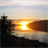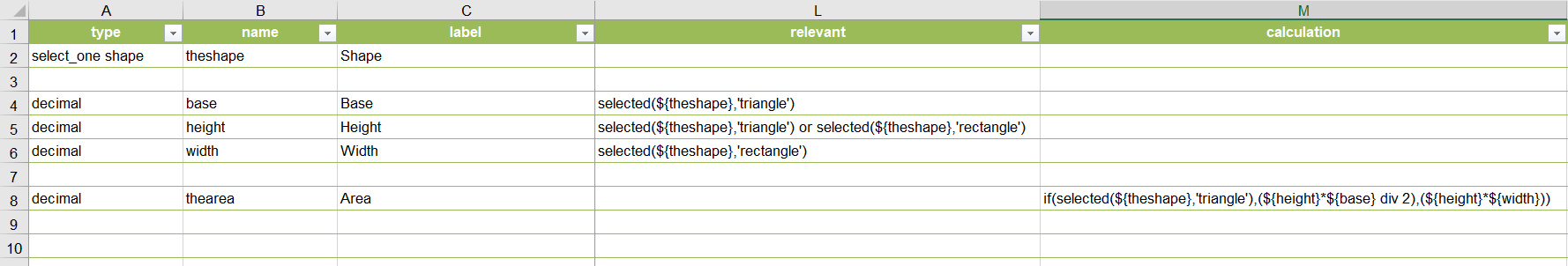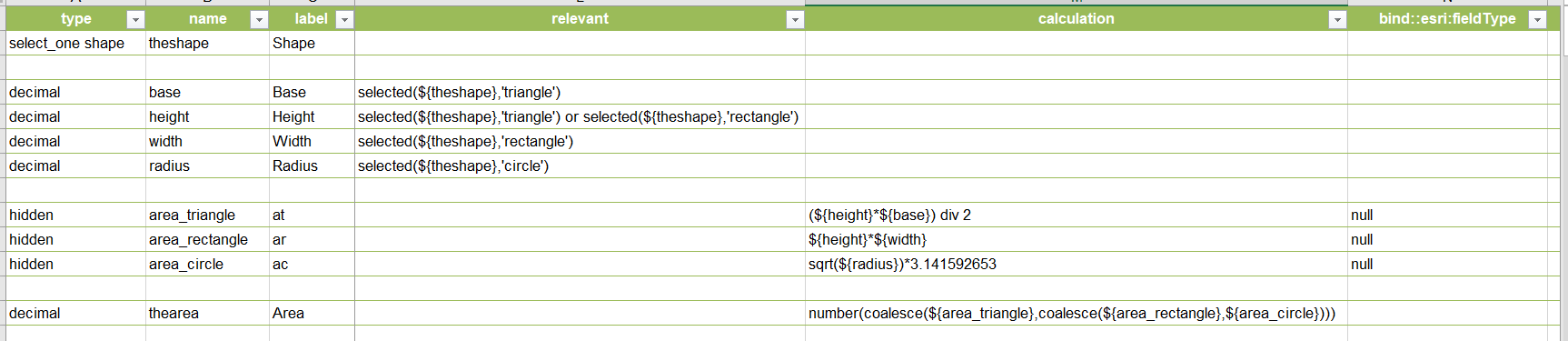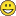# Multiple Calculation Options for one Field Survey123?

591
3
04-07-2020 07:11 AMNew Contributor III

Hey everybody,

Background:
I feel like I am always trying to push the limitations of Survey123, what a fun time. Anyways, I have created a Survey form for recording Water Breaks (Leaks). I have used "Constraints" to allow certain variables for the calculations I need to appear based on the type of water break (hole, rectangular, circular are my 3 options). So if the field worker clicks rectangular break, then they will have the option to enter in the length, width etc.. needed to perform the calculcation.

My question:

I have one field for the "Area" of the water leak break needed for me to do calculate water loss. All 3 water break types share this value, but use different calculations to achieve this value. Is there a way I can put multiple equations in the field but only using the desired equation (say IF "Rectangular_Break" is chosen use this equation OR IF "Circular_Break" is chosen use this equation.

Possible Solution

My only thought, which is not ideal, but is it use multiple "Area" fields based on the break type, but then for my next calculations I would have to make multiple "Water loss calculations" etc.. based on the break type. If that makes sense. I was thinking if Repeats or IF statement etc.. can be used with calculations could create a work around?

Thank you all, if you need anything to help figure out what I am trying to achieve please comment. I am attaching the water breaks equations that I am basing my survey123 off of (you can see they share variables etc..). I can share my survey too but would prefer to DM. Appreciate it!

Tags (2)
1 Solution

Accepted SolutionsbyEsri Notable Contributor

Try this branching out your calculation using if. As shown in the simplified example below.

The if function, takes three parameters: 1) A function to evaluate. 2) A function to provide an output if expression 1 is true, 2) A function to provide an output if expression is false.3 RepliesbyEsri Notable Contributor

Try this branching out your calculation using if. As shown in the simplified example below.

The if function, takes three parameters: 1) A function to evaluate. 2) A function to provide an output if expression 1 is true, 2) A function to provide an output if expression is false.byEsri Notable Contributor

Here is something even simpler.New Contributor III

Hey Ismael,

The If function worked great, I couldn't get the Coalesce to work with my calculation for some reason (probably did something wrong, will probably try that again separately just for fun).

Thanks for everything you and James Tedrick‌ do; really been liking incorporating Survey123 with Collector